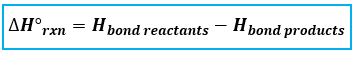# Problem: In the Chemistry and the Environment box on free radicals in Chapter 9 in the textbook, we discussed the importance of the hydroxyl radical in reacting with and eliminating many atmospheric pollutants. However, the hydroxyl radical does not clean up everything. For example, chlorofluorocarbons-which destroy stratospheric ozone-are not attacked by the hydroxyl radical. Consider the hypothetical reaction by which the hydroxyl radical might react with a chlorofluorocarbon: OH(g) + CF2Cl2(g) → HOF(g) + CFCl2 (g). Use bond energies to explain why this reaction is improbable.

###### FREE Expert Solution

To calculate the ΔH°rxn using bond energies, we’re going to use the following equation:Let’s first figure out what kind of bonds and how many moles are present in each reactants and products because the given bond energies are per mole.

Balanced Reaction: OH(g) + CF2Cl2(g) → HOF(g) + CFCl2 (g)

*always make sure that the given reaction is balanced###### Problem Details

In the Chemistry and the Environment box on free radicals in Chapter 9 in the textbook, we discussed the importance of the hydroxyl radical in reacting with and eliminating many atmospheric pollutants. However, the hydroxyl radical does not clean up everything. For example, chlorofluorocarbons-which destroy stratospheric ozone-are not attacked by the hydroxyl radical. Consider the hypothetical reaction by which the hydroxyl radical might react with a chlorofluorocarbon: OH(g) + CF2Cl2(g) → HOF(g) + CFCl2 (g). Use bond energies to explain why this reaction is improbable.